# # 教你用 IDA

## # IDA 迷惑代码大赏

1. 首先介绍一下 IDA 里常见的屎，我们打开一道某城杯的 ez_linklist 题目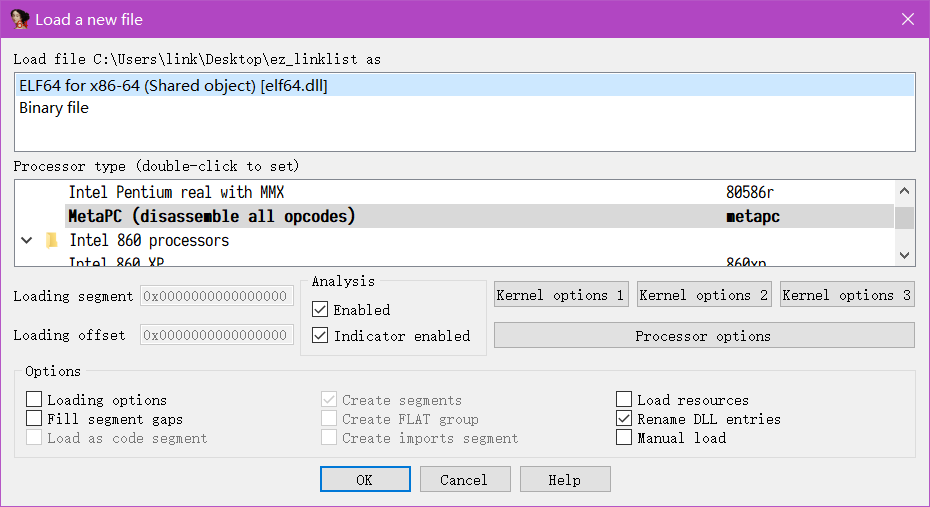1. 点下 ok 找到 main 函数，映入眼帘就是一坨很经典的屎， `switch跳转表识别错误` ，属于是史里比较正常的一坨，这还不是很影响分析的部分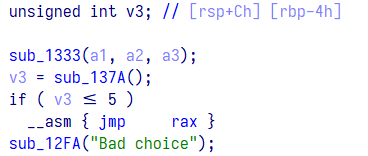1. 看一眼侧边栏，很经典的 `拿掉自定义的函数名` ，那么本程序的数据结构肯定也就没有了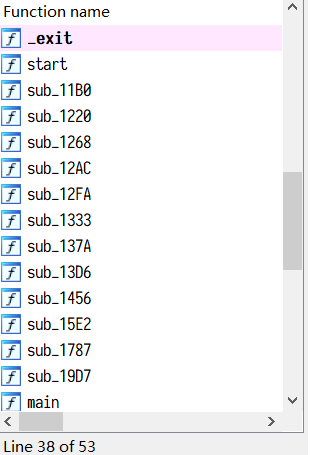1. 我们向上逐个点进去看一眼，更是个寄吧， `各种解引用强转` 写的和一把米诺一样（恼），看起来就像是精神状态欠佳的人写出来的代码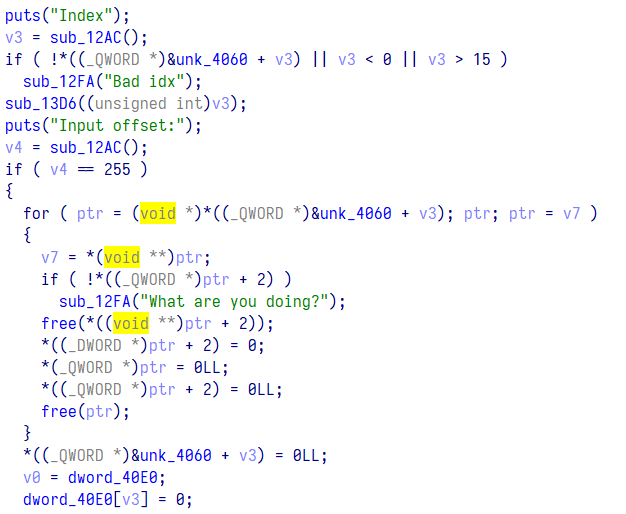1. 接下来点进 puts 下面的函数， `call了不知道个什么b玩意` ，鉴定为纯纯的纯纯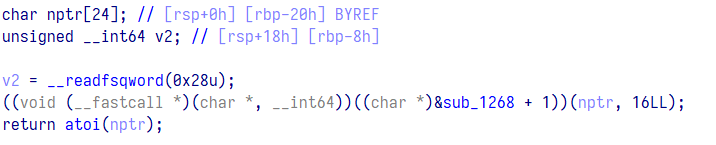1. 这里面更是直接 `见红`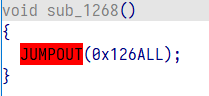## # C 语言变量复习

``````int a;
int b;``````

a 便是 int 类型的变量，b 是一个 int 数组，他们都是我们的常见类型

1. 其中 a 实际上指向了一块地址所指的值，其 int 表示将 a 地址中的东西以 int 类型来解析出来，所以我们直接输出 a 可以输出 `a 存放的值`

2. 而 b 指向了一块地址，其 int 表示 将 b 里面的数据用 int 类型解析出来，但是直接输出 b 不能输出 `b 存放的值` ，而是输出了 `b 的地址` ，因为 `b` 本身就是一个地址，因此需要加上 b 后面的下标也就是 b [x] , 才能输出 `b 对应位置所存放的值` ，对 b 取索引的操作也同样是 `对 b 以 int 类型解引用`

`a的地址所指向的值` 被 c 语言直接当成了 a 的值 。

`b的地址` 被 c 语言当成了 b 的值，因此，我们也将 b 称之为指针

``````#include <stdio.h>
#include <stdlib.h>

void main()
{
int a = 114514;
int b = {114514,1919};
printf("%d\n" , a);
printf("%d\n" , b);
}``````

``````114514
469523392  以16进制表示 0x1bfc5bc0``````

``````#include <stdio.h>
#include <stdlib.h>

void main()
{
int a = 114514;
int b = {114514 , 1919};
printf("%d\n" , a);
printf("%d\n" , b);

printf("%d\n" , *b);
}``````

``````114514
-879752048
114514``````

``````#include <stdio.h>
#include <stdlib.h>

void main()
{
int a = 114514;
int b = {114514 , 1919};
printf("%d\n" , a);
printf("%d\n" , b);

printf("%d\n" , *b);
printf("%d\n" , *(b+1));
}``````

``````114514
289332624
114514
1919``````

``````#include <stdio.h>
#include <stdlib.h>

void main()
{
int a = 114514;
int b = {114514 , 1919};
printf("%d\n" , a);
printf("%d\n" , b);

printf("%d\n" , *b);
printf("%d\n" , *(b+1));
printf("%d\n" , *(int*)((void *)b+4) ) ;
}``````

``````114514
1692555520
114514
1919
1919``````

a = b[-1] = *b-1 = *(int *)b-1 = *(int *)((void *)b-4)

``````#include <stdio.h>
#include <stdlib.h>

void main()
{
int a = 114514;
int b = {114514 , 1919};
printf("%d\n" , a);
printf("%d\n" , b);

printf("%d\n" , *b);
printf("%d\n" , *(b+1));

printf("%d\n" , *(int*)((void *)b+4) ) ;
printf("%d\n" , &a);
printf("%d\n" , *&a);
}``````

``````114514
1006296128
114514
1919
1919
1006296124
114514``````

## # 把史包装成一坨能上餐桌的史

### # 修复错误识别

``((void (__fastcall *)())((char *)&sub_1268 + 1))();``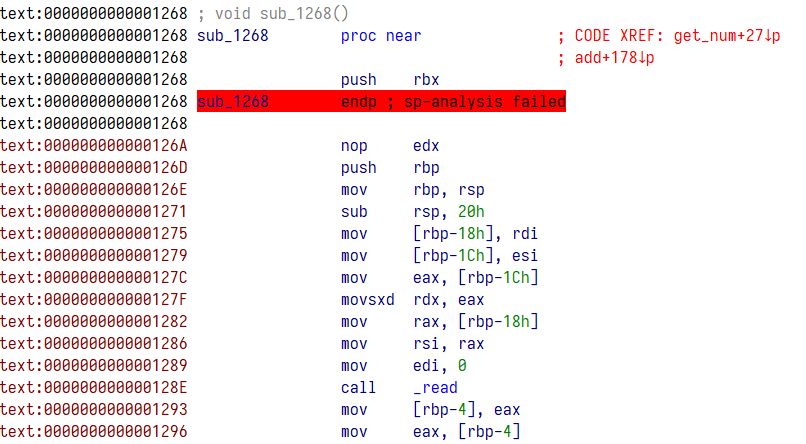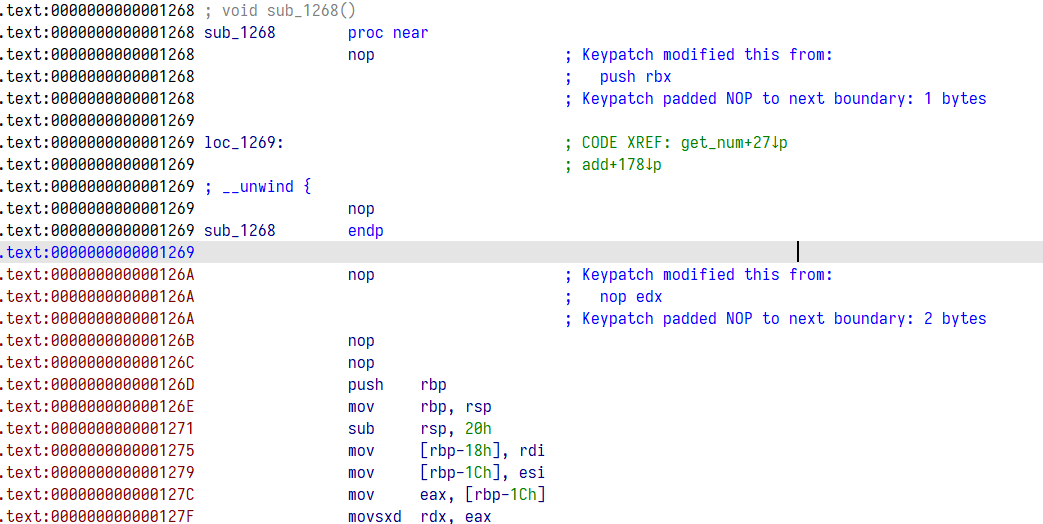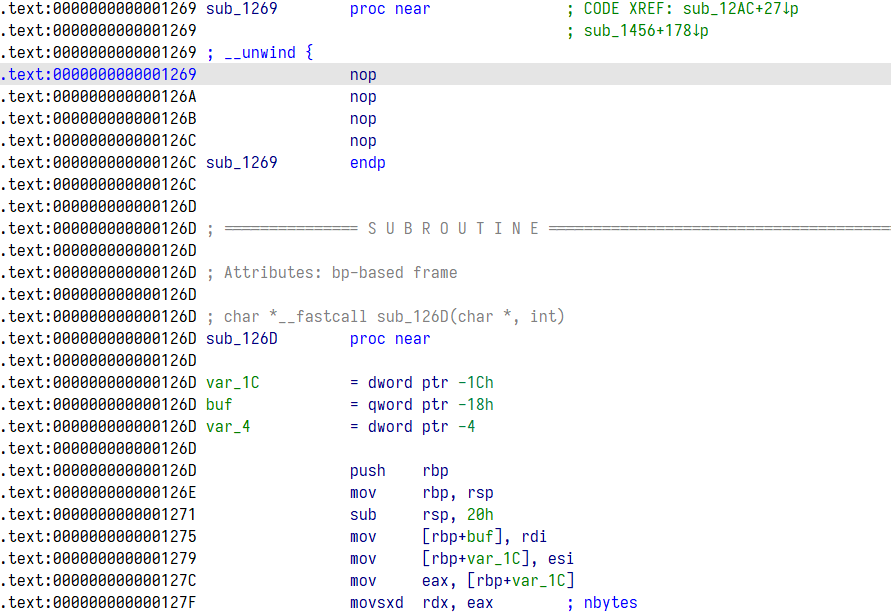### # 修复 switch 跳转表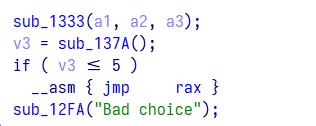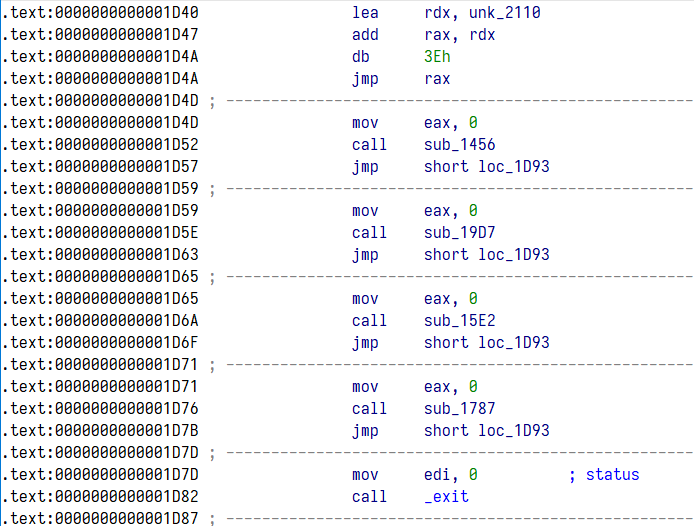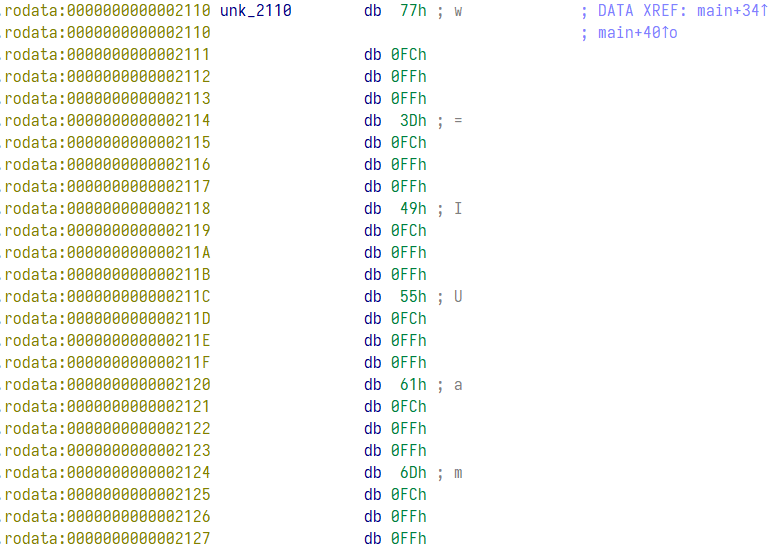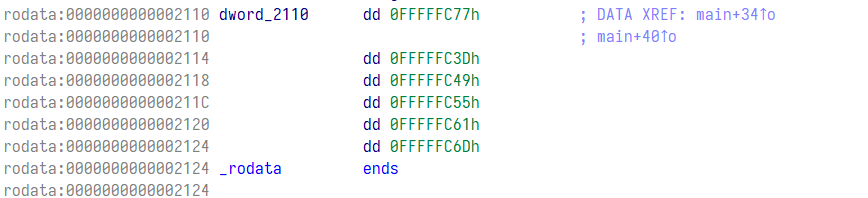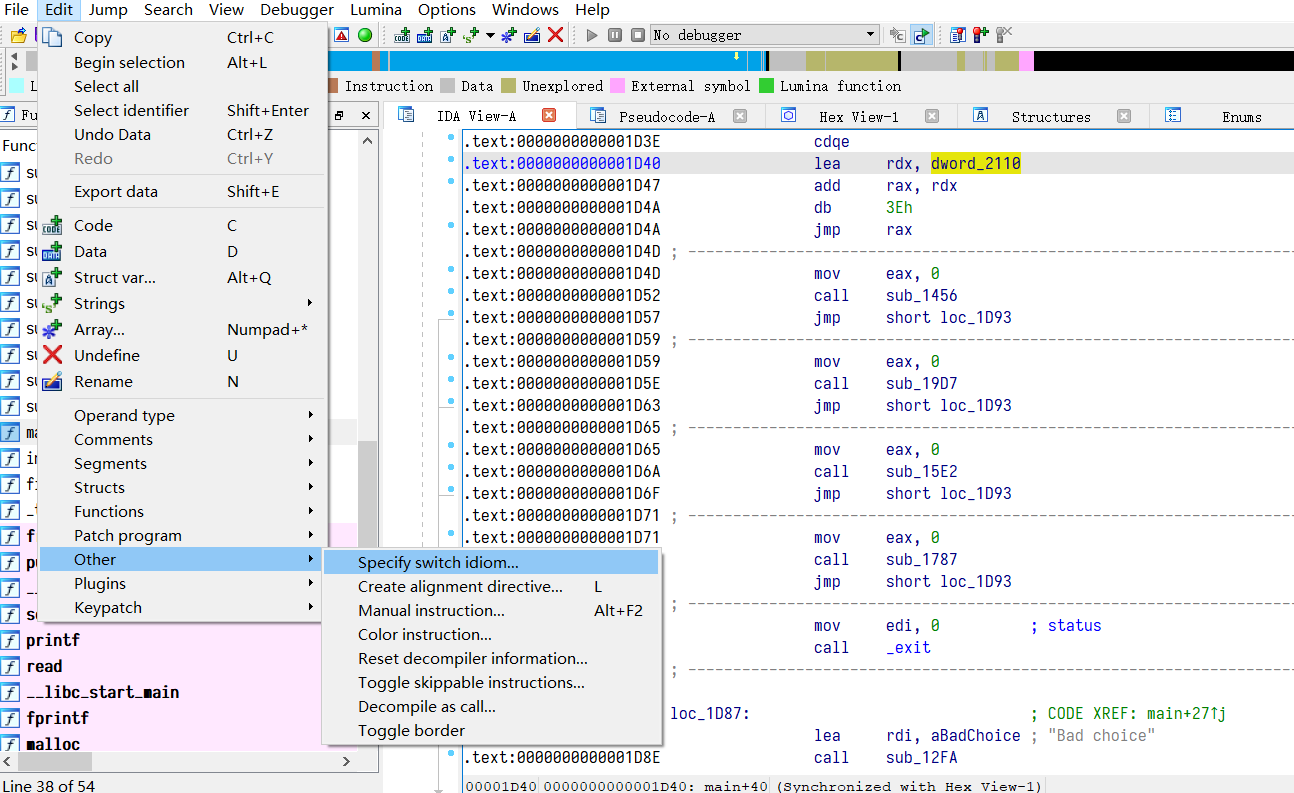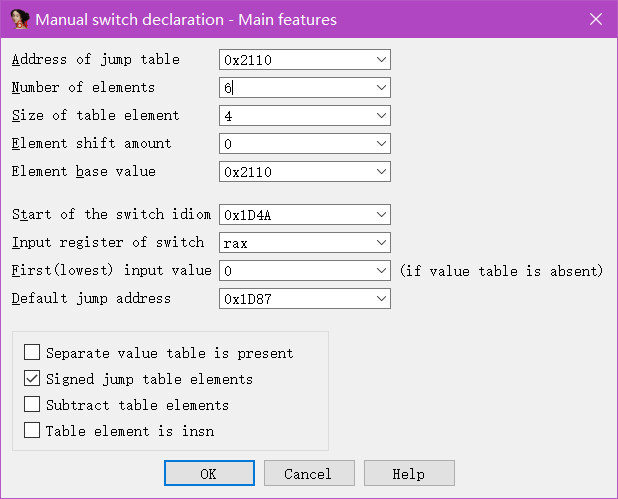Number of elements ：跳转元素个数，这个例子是 6

Size of table element ：跳转表每个元素长度，这里是 4

Element base value ：这里和第一个相同即可

Input register of switch ：跳转到的寄存器，这里是 rax

Signed jump table elements ：如果上面看到的跳转表是负数则需要勾选此选项，比如之前看到的数据是 0xff 开头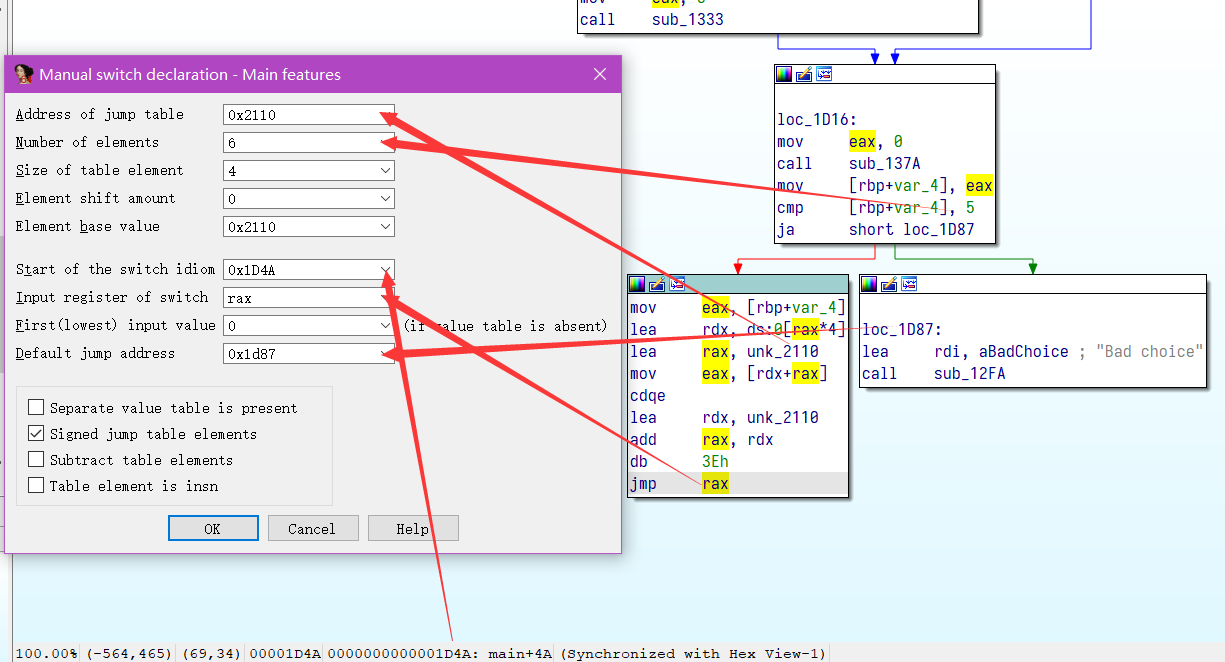IDA 修复跳表_huzai9527 的博客 - CSDN 博客_ida switch 修复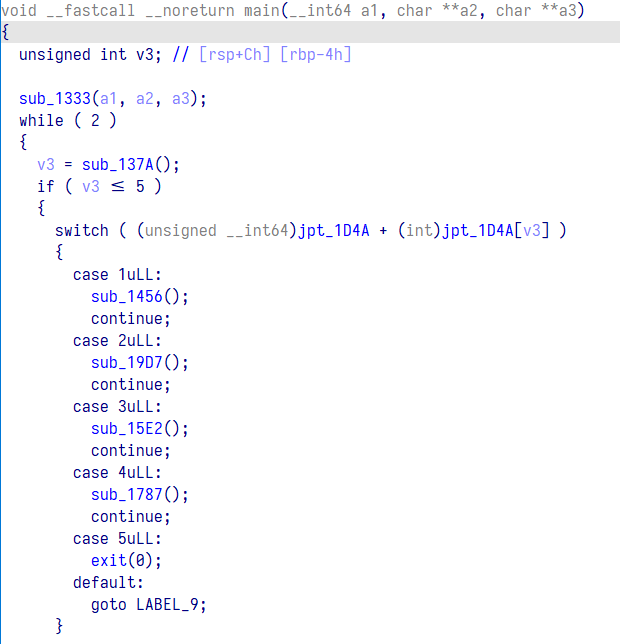### # 重命名函数和变量

ida `快捷键 n` ， 在变量名或者函数名处按 n 便可以对函数进行重命名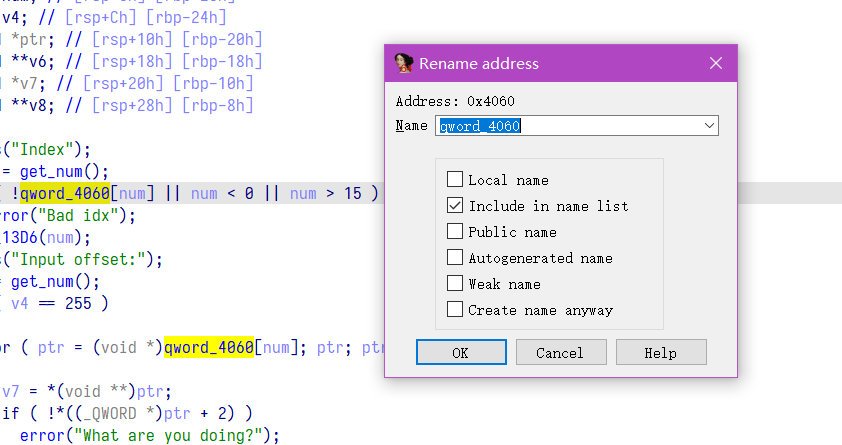``````int add()
{
int i; // [rsp+0h] [rbp-10h]
int choice; // [rsp+4h] [rbp-Ch]
void *buf; // [rsp+8h] [rbp-8h]

for ( i = 0; i <= 15 && *((_QWORD *)&ptr_list + i); ++i )
;
if ( i > 15 )
puts("Size:");
choice = get_num();
if ( choice > 0x70 || choice < 0 )
error("wrong size!");
buf = malloc(choice);
*((_QWORD *)&ptr_list + i) = malloc(0x18uLL);
*(_DWORD *)(*((_QWORD *)&ptr_list + i) + 8LL) = choice;
*(_QWORD *)(*((_QWORD *)&ptr_list + i) + 16LL) = buf;
**((_QWORD **)&ptr_list + i) = 0LL;
count[i] = 1;
puts("Content:");
return puts("Success");
}``````

### # 修复强制转换

``*((_QWORD *)&ptr_list + i)``

ida `快捷键 y` ， 在变量名或者函数名处按 y 便可以对函数进行类型修改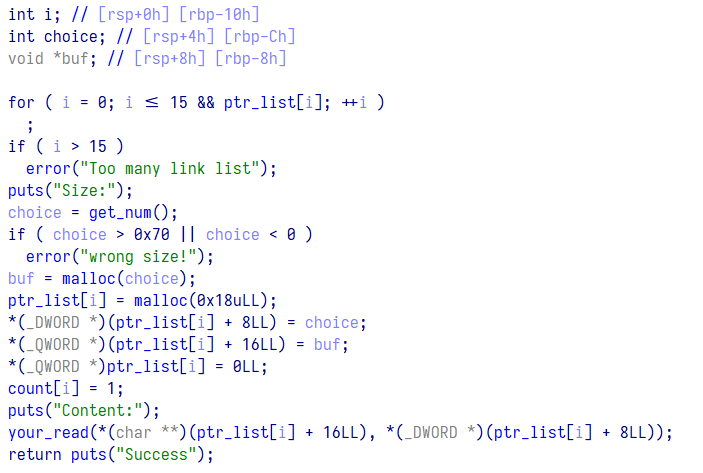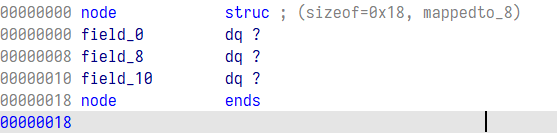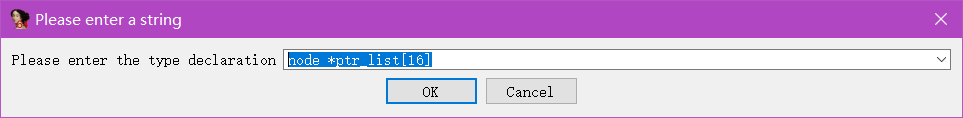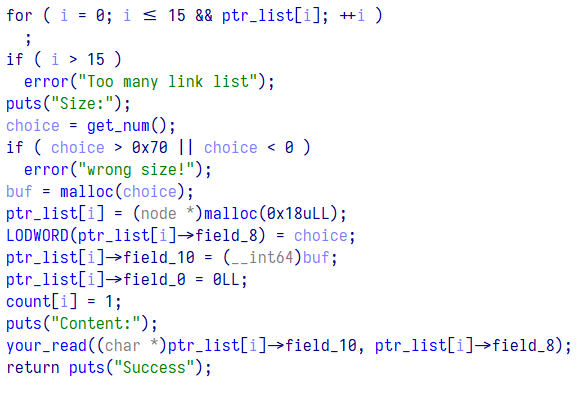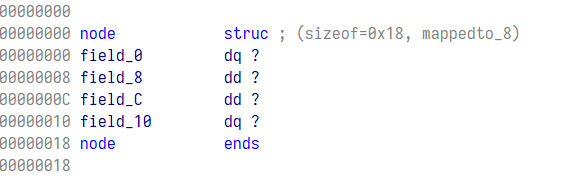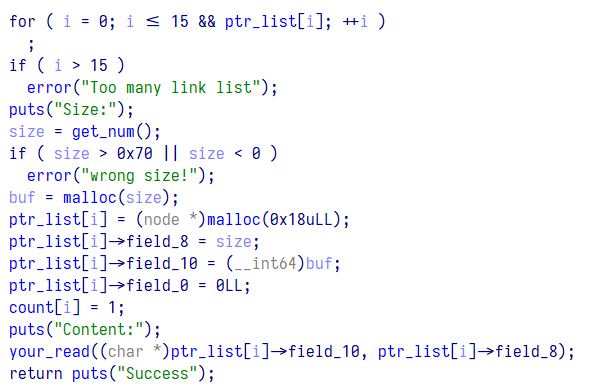``````int add()
{
int i; // [rsp+0h] [rbp-10h]
int size; // [rsp+4h] [rbp-Ch]
char *buf; // [rsp+8h] [rbp-8h]

for ( i = 0; i <= 15 && ptr_list[i]; ++i )
;
if ( i > 15 )
puts("Size:");
size = get_num();
if ( size > 0x70 || size < 0 )
error("wrong size!");
buf = (char *)malloc(size);
ptr_list[i] = (node *)malloc(0x18uLL);
ptr_list[i]->size = size;
ptr_list[i]->data = buf;
ptr_list[i]->field_0 = 0LL;
count[i] = 1;
puts("Content:");
return puts("Success");
}``````

``````int link()
{
int i; // [rsp+Ch] [rbp-14h]
int idx1; // [rsp+10h] [rbp-10h]
int idx2; // [rsp+14h] [rbp-Ch]
node *ptr; // [rsp+18h] [rbp-8h]

idx1 = get_num();
if ( !ptr_list[idx1] || idx1 < 0 || idx1 > 15 )
idx2 = get_num();
if ( !ptr_list[idx2] || idx2 < 0 || idx2 > 15 )
ptr = ptr_list[idx1];
for ( i = 1; i < count[idx1]; ++i )
ptr = (node *)ptr->field_0;
ptr->field_0 = (__int64)ptr_list[idx2];
count[idx1] += count[idx2];
count[idx2] = 0;
ptr_list[idx2] = 0LL;
return puts("Success!");
}``````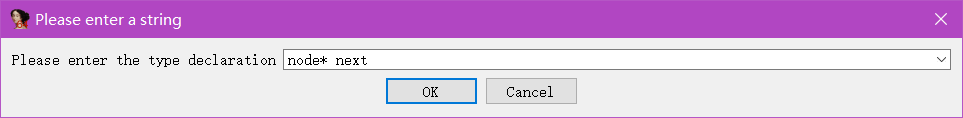## # 高质量代码鉴赏环节

### # main

``````void __fastcall __noreturn main(__int64 a1, char **a2, char **a3)
{
unsigned int choice; // [rsp+Ch] [rbp-4h]

setvbuf();
while ( 2 )
{
if ( choice <= 5 )
{
switch ( (unsigned __int64)table + (int)table[choice] )
{
case 1uLL:
continue;
case 2uLL:
delete();
continue;
case 3uLL:
continue;
case 4uLL:
continue;
case 5uLL:
exit(0);
default:
goto LABEL_9;
}
}
break;
}
LABEL_9:
}``````

``````int add()
{
int i; // [rsp+0h] [rbp-10h]
int size; // [rsp+4h] [rbp-Ch]
char *buf; // [rsp+8h] [rbp-8h]

for ( i = 0; i <= 15 && ptr_list[i]; ++i )
;
if ( i > 15 )
puts("Size:");
size = get_num();
if ( size > 0x70 || size < 0 )
error("wrong size!");
buf = (char *)malloc(size);
ptr_list[i] = (node *)malloc(0x18uLL);
ptr_list[i]->size = size;
ptr_list[i]->data = buf;
ptr_list[i]->next = 0LL;
count[i] = 1;
puts("Content:");
return puts("Success");
}``````
``````int link()
{
int i; // [rsp+Ch] [rbp-14h]
int idx1; // [rsp+10h] [rbp-10h]
int idx2; // [rsp+14h] [rbp-Ch]
node *ptr; // [rsp+18h] [rbp-8h]

idx1 = get_num();
if ( !ptr_list[idx1] || idx1 < 0 || idx1 > 15 )
idx2 = get_num();
if ( !ptr_list[idx2] || idx2 < 0 || idx2 > 15 )
ptr = ptr_list[idx1];
for ( i = 1; i < count[idx1]; ++i )
ptr = ptr->next;
ptr->next = ptr_list[idx2];
count[idx1] += count[idx2];
count[idx2] = 0;
ptr_list[idx2] = 0LL;
return puts("Success!");
}``````
``````int unlink()
{
int i; // [rsp+8h] [rbp-18h]
int j; // [rsp+Ch] [rbp-14h]
int idx; // [rsp+10h] [rbp-10h]
int offset; // [rsp+14h] [rbp-Ch]
node *ptr; // [rsp+18h] [rbp-8h]

for ( i = 0; i <= 15 && ptr_list[i]; ++i )
;
if ( i > 15 )
puts("Index:");
idx = get_num();
if ( !ptr_list[idx] || idx < 0 || idx > 15 )
if ( count[idx] == 1 )
error("This list has only one node.");
check(idx);
puts("Input offset:");
offset = get_num();
if ( offset >= count[idx] || offset < 0 )
if ( offset )
{
ptr = ptr_list[idx];
for ( j = 0; j < offset - 1; ++j )
ptr = ptr->next;
ptr_list[i] = ptr->next;
ptr->next = ptr->next->next;
}
else
{
ptr_list[i] = ptr_list[idx];
ptr_list[idx] = ptr_list[idx]->next;
}
--count[idx];
count[i] = 1;
return puts("Success");
}``````

### # delete

``````int delete()
{
_DWORD *res; // rax
int i; // [rsp+4h] [rbp-2Ch]
int idx; // [rsp+8h] [rbp-28h]
int offset; // [rsp+Ch] [rbp-24h]
node *ptr; // [rsp+10h] [rbp-20h]
node *p; // [rsp+18h] [rbp-18h]
node *next; // [rsp+20h] [rbp-10h]
node *buf; // [rsp+28h] [rbp-8h]

puts("Index");
idx = get_num();
if ( !ptr_list[idx] || idx < 0 || idx > 15 )
check(idx);
puts("Input offset:");
offset = get_num();
if ( offset == 0xFF )
{
for ( ptr = ptr_list[idx]; ptr; ptr = next )
{
next = ptr->next;
if ( !ptr->data )
error("What are you doing?");
free(ptr->data);
ptr->size = 0;
ptr->next = 0LL;
ptr->data = 0LL;
free(ptr);
}
ptr_list[idx] = 0LL;
res = count;
count[idx] = 0;
}
else
{
if ( offset >= count[idx] || offset < 0 )
p = ptr_list[idx];
if ( offset )
{
for ( i = 0; i < offset - 1; ++i )
p = p->next;
buf = p->next;
p->next = p->next->next;
if ( !buf->data )
error("What are you doing?");
free(buf->data);
free(buf);
}
else
{
ptr_list[idx] = ptr_list[idx]->next;
if ( !p->data )
error("What are you doing?");
free(p->data);
p->next = 0LL;
p->size = 0;
p->data = 0LL;
free(p);
}
if ( !--count[idx] )
ptr_list[idx] = 0LL;
LODWORD(res) = puts("Success");
}
return (int)res;
}``````